Published

Plant Babysitter

Never kill a plant again with this hands-free plant babysitter that quenches your plant's thirst so you don't have to!

BeginnerFull instructions provided896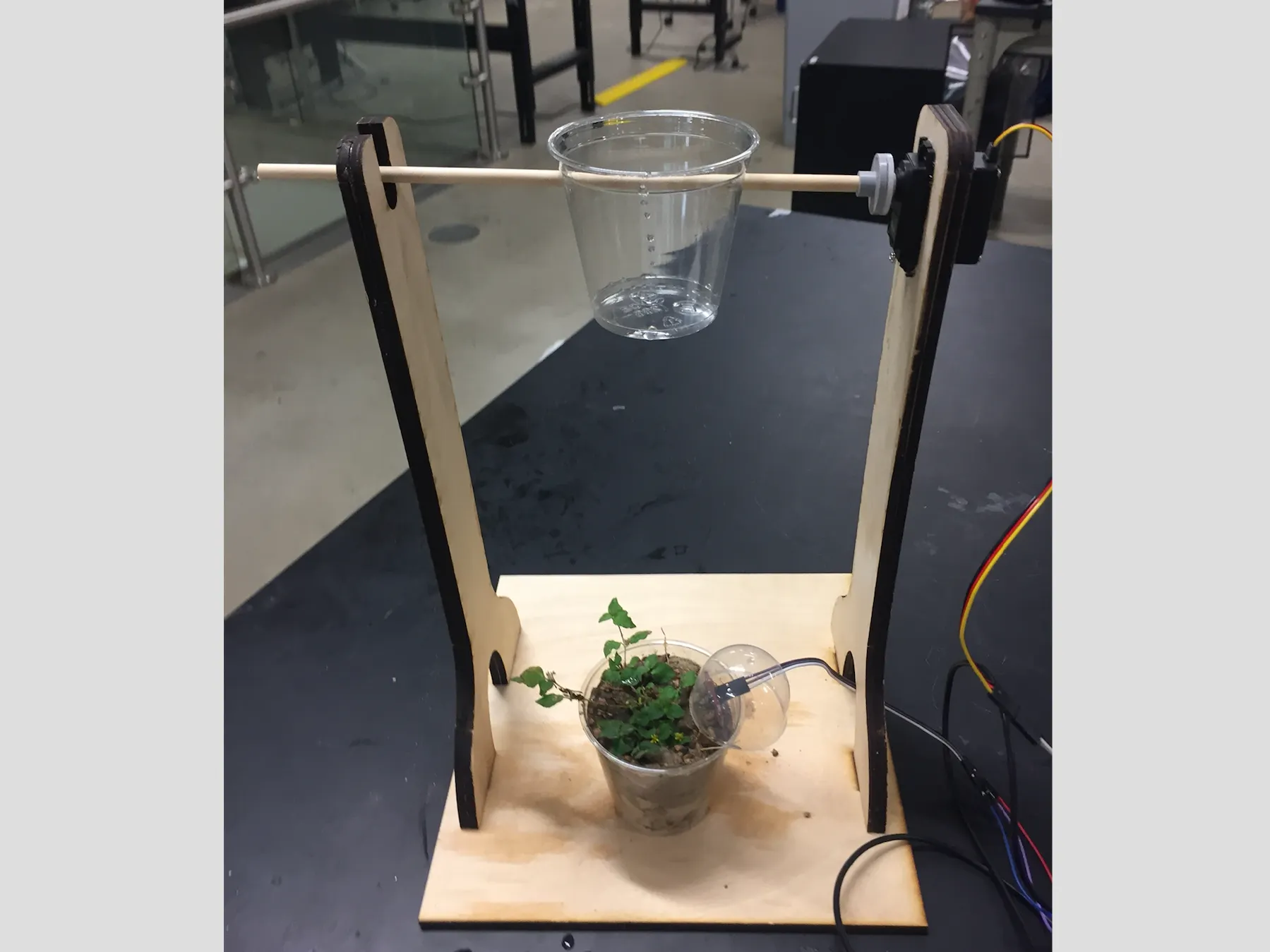Things used in this project

Hardware components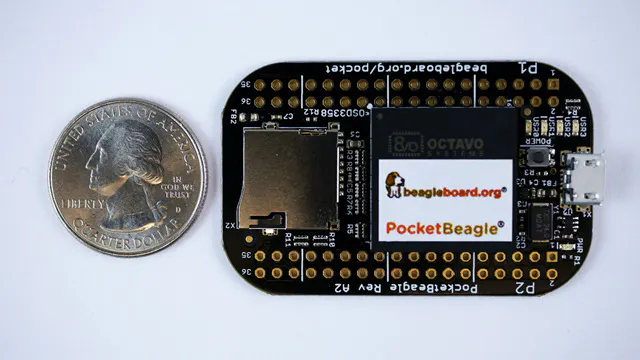BeagleBoard.org PocketBeagle
×1
 Servos (Tower Pro MG996R)
×1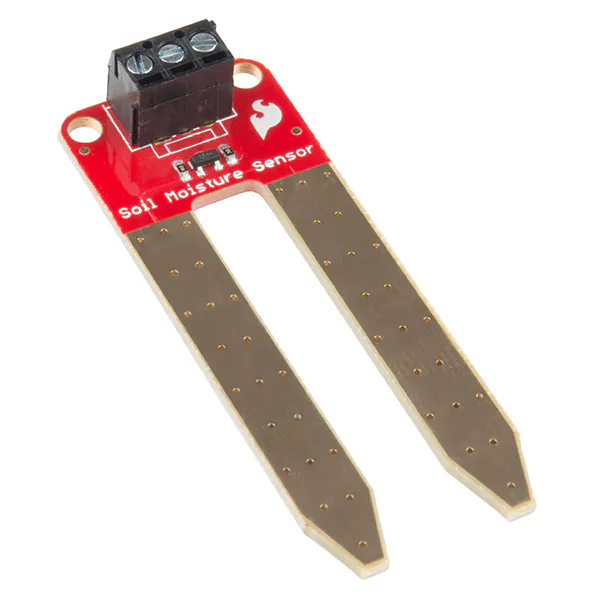SparkFun Soil Moisture Sensor (with Screw Terminals)
×1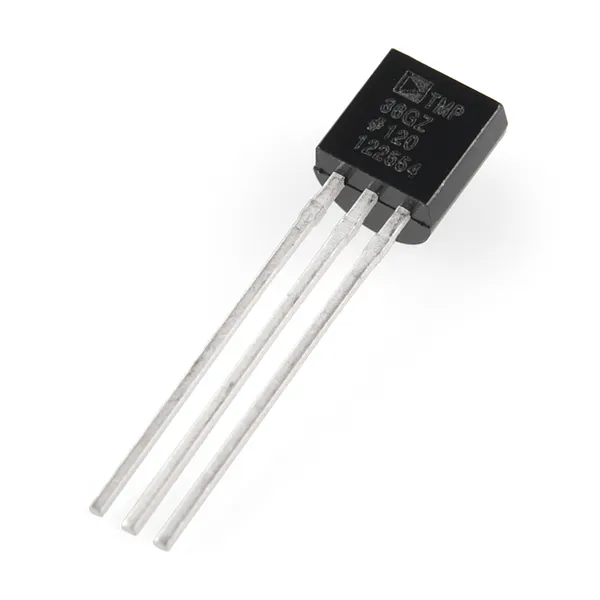Temperature Sensor
×1Jumper wires (generic)
×1×1

Hand tools and fabrication machinesLaser cutter (generic)3D Printer (generic)

Schematics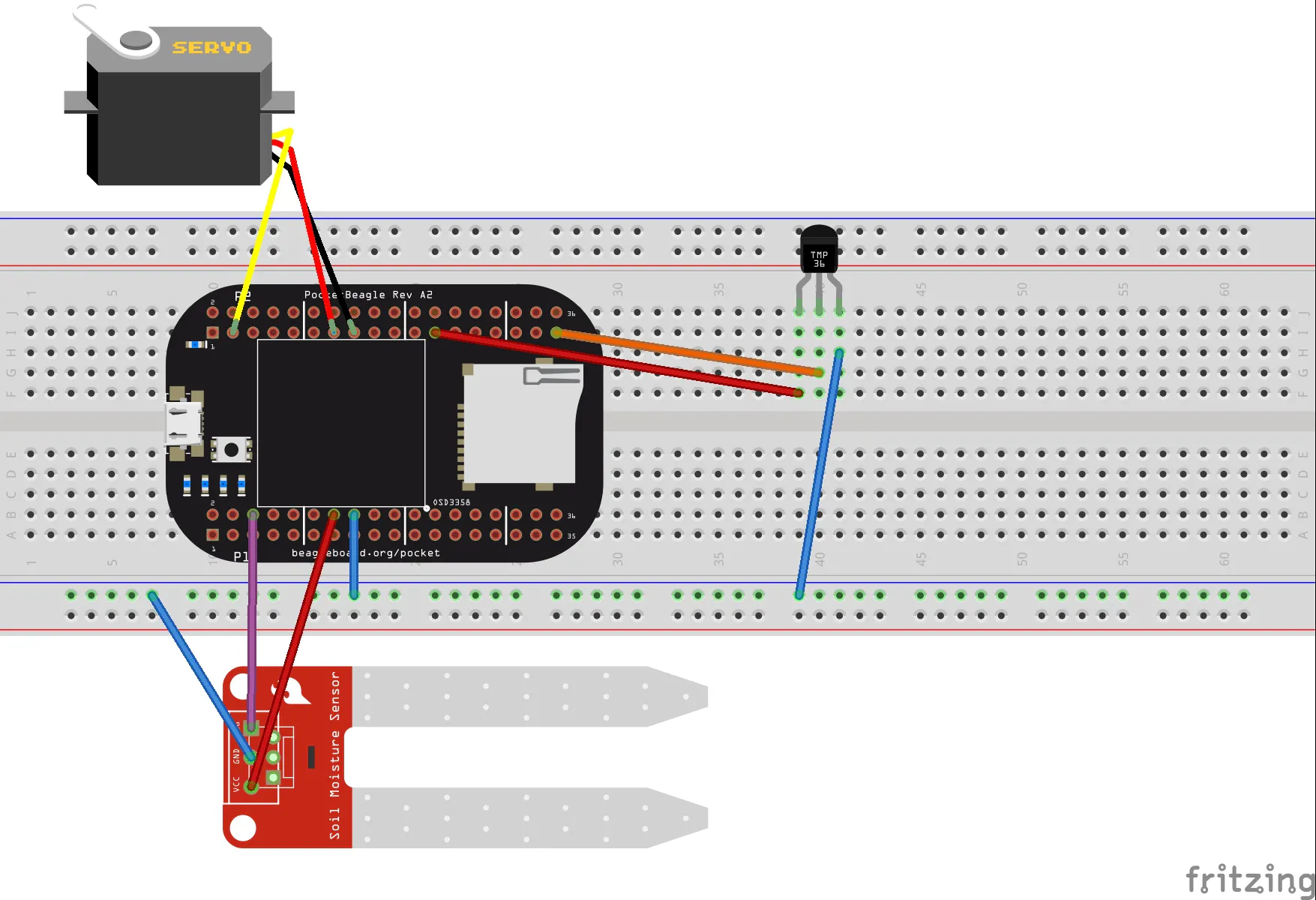Code

Plant Babysitter Code

Python
This code checks the moisture, reads the temperature, and waters the plant if necessary. The program will continue to run until you exit it (cntrl c). You may have to run the code using sudo.
# ------------------------------------------------------------------------
# Constants
# ------------------------------------------------------------------------
import time
# Peripheral path
GPIO_BASE_PATH               = "/sys/class/gpio" #sets up path to get to file
ADC_BASE_PATH                = "/sys/bus/iio/devices/iio:device0" #sets up path to get to file

# GPIO direction
IN                           = True #Gpio is receiving input (we don't have this)
OUT                          = False #Gpio is outputting (this is what we have)

# GPIO output state
LOW                          = "0" #basically off
HIGH                         = "1" #basically on

GPIO_VAL                     = (0, 5)  # gpio5 in tupple form

# Moisture Sensor ADC input
SensorReading                = "in_voltage6_raw" # AIN6   (this is the file name)
TempReading                  = "in_voltage5_raw" # AIN5

# ------------------------------------------------------------------------
# FUNCTIONS
# ------------------------------------------------------------------------
import os

def gpio_setup(gpio, direction, default_value=False):
"""Setup GPIO pin

* Test if GPIO exists; if not create it
* Set direction
* Set default value
"""
#This is borrowed from whac-a-mole code

gpio_number = str((gpio * 32) + gpio)
path        = "{0}/gpio{1}".format(GPIO_BASE_PATH, gpio_number)

if not os.path.exists(path):
# "echo {gpio_number} > {GPIO_BASE_PATH}/export"
print("Create GPIO: {0}".format(gpio_number))
with open("{0}/export".format(GPIO_BASE_PATH), 'w') as f:
f.write(gpio_number)

if direction:
# "echo in > {path}/direction"
with open("{0}/direction".format(path), 'w') as f:
f.write("in")
else:
# "echo out > {path}/direction"
with open("{0}/direction".format(path), 'w') as f:
f.write("out")

if default_value:
# "echo {default_value} > {path}/value"
with open("{0}/value".format(path), 'w') as f:
f.write(default_value)
# End def

def gpio_set(gpio, value):
"""Set GPIO ouptut value."""
gpio_number = str((gpio * 32) + gpio)
path        = "{0}/gpio{1}".format(GPIO_BASE_PATH, gpio_number)

# "echo {value} > {path}/value"
with open("{0}/value".format(path), 'w') as f:
f.write(value)
# End def

"""Get ADC input value.
Returns:
value (float):  Value will be between 0 (0V) and 1.0 (3.3V)."""
#channel is the adc raw input that we defined as SensorReading
with open("{0}/{1}".format(ADC_BASE_PATH, channel), 'r') as f:

return float(float(out) / float(4096)) #returns the analog number in decimal form (between 0 and 1)
# End def

def turn_off_moisture_sensor():
"""Turn off the moisture sensor
"""
gpio_set(GPIO_VAL, LOW)
# End def

def turn_on_moisture_sensor():
"""Turn on the moisture sensor
"""
gpio_set(GPIO_VAL, HIGH)
# End def

def setup_sensor():
"""Setup all the pins for the reading.
"""
gpio_setup(GPIO_VAL, OUT, LOW)
#End def

# ------------------------------------------------------------------------
# SEMI-DRIVERS?
# ------------------------------------------------------------------------

""" set up stuff and read moisture level"""
setup_sensor()
turn_on_moisture_sensor()
turn_off_moisture_sensor()
return moisture

#End def

def water_plant():
"""activates the servo motor to water the plant
"""
import Adafruit_BBIO.PWM as PWM
import time

servo_pin = "P2_3"
duty_min = 3
duty_max = 14.5
duty_span = duty_max - duty_min

PWM.start(servo_pin, (100-duty_min), 20.0)
duty = 4
PWM.set_duty_cycle(servo_pin, duty)
time.sleep(1.0)

PWM.stop(servo_pin)
PWM.cleanup()
time.sleep(2.0)

PWM.start(servo_pin, (100-duty_min), 20.0)
duty = 1.5
PWM.set_duty_cycle(servo_pin, duty)
time.sleep(1.0)

PWM.stop(servo_pin)
PWM.cleanup()

#End def

def setup():
setup_sensor()
#End def

tempMV = tempAnalog * 3300
temp_c = (tempMV - 500) / 10
temp_f = (temp_c * 9/5) + 32
temperature = ('C=%d F=%d' % (temp_c, temp_f))
return temperature
time.sleep(1)

return temperature
#End def

# ------------------------------------------------------------------------
# MAIN DRIVER
# ------------------------------------------------------------------------
def main():
setup()

while (True):
print("Temperature: {0}".format(temperature))
print("Moisture Level = {0}".format(moisture))
if (moisture < 0.5):
print("Moisture low, watering plant")
water_plant()
else:
print("All is well!")

time.sleep(60.0) #sleeps for 1 minute
#End def

main()

Credits

Serena Agrawal

1 project • 1 follower
Rice University 2019, Mechanical Engineering